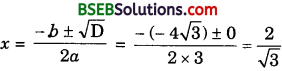# Bihar Board Class 10th Maths Solutions Chapter 4 Quadratic Equations Ex 4.4

Bihar Board Class 10th Maths Solutions Chapter 4 Quadratic Equations Ex 4.4 Textbook Questions and Answers.

## BSEB Bihar Board Class 10th Maths Solutions Chapter 4 Quadratic Equations Ex 4.4

Question 1.
Find the nature of the roots of the following quadratic equations. If the real roots exist, find them:
(i) 2x2 – 3x + 5 = 0
(ii) 3x2 – 4$$\sqrt{3}$$x + 4 = 0
(iii) 2x2 – 6x + 3 = 0
Solution:
(i) The given equation is 2x2 – 3x + 5 = 0.
Here, a = 2, b = – 3 and c = 5.
∴ D = b2 – 4ac = (- 3)2 – 4 × 2 × 5 = 9 – 40 = – 31 < 0
So, the given equation has no real roots.

(ii) The given’equation is 3x2 – 4$$\sqrt{3}$$x + 4 = 0
Here, a = 3, b = – 4$$\sqrt{3}$$ and c = 4.
∴ D = b2 – 4ac = (- 4$$\sqrt{3}$$)2 – 4 × 2 × 5 = 9 – 40 = – 31 < 0
So, the given equation has real equal roots, given by(iii) The given equation is 2x2 – 6x + 3 = 0.
Here, a = 2, b = – 6 and c = 3
∴ D = b2 – 4ac = (- 6)2 – 4 × 2 × 3 = 36 – 24 = 12 > 0
So, the given equation has real roots, given by∴ The roots of the given equation are $$\frac{3+\sqrt{3}}{2}$$ and $$\frac{3-\sqrt{3}}{2}$$.Question 2.
Find the values of k for each of the following quadratic equations, so that they have two equal roots:
(i) 2x2 + kx + 3 = 0
(ii) kx(x – 2) + 6 = 0
Solution:
(i) The given equation is 2x2 + kx + 3 = 0.
Here, a = 2, b = k and c = 3
∴ D = b2 – 4ac = k2 – 4 × 2 × 3 = k2 – 24
The given equation will have real and equal roots, if
D = 0 i.e; k2 – 2kx + 6 = 0
Here, a = k, b = – 2k and c = 6
∴ D = b2 – 4ac = (- 2k)2 – 4 × k × 6 = 4k2 – 24k
The given equation will have real and equal roots, if D = 0.
So, 4k2 – 24k = 0 or 4k(k – 6) = 0
i.e., k = 0 or k = 6.

Question 3.
Is it possible to design a rectangular mango grove whose length is twice its breadth, and the area is 800 m2? If so, find its length and breadth.
Solution:
Let 2x be the length and x be the breadth of a rectangular mango grove.
Area = (2x)(x) = 800. [Given]
So, x2 = 400 or x = 20 [Since side cannot be negative]
The value of x is real so to design of grove is possible
Its length = 40 m and breadth = 20 m.Question 4.
Is the following situation possible? If so, determine their present ages. The sum of the ages of two friends is 20 years. Four years ago, the product of their ages in years was 48.
Solution:
Let age of one of the friends = x years. Then, age of the other friend = 20 – x.
Four years ago,
Age of one of the friends = (x – 4) years
and age of the other friend = (20 – x – 4) years
= (16 – x) years

According to condition:
(x – 4)(16 -x) = 48
or 16x – x2 – 64 + 4x = 48
or x2 – 20x + 112 = 0
Here, a = 1, b = – 20 and c = 112.
∴ D = b2 – 4ac = (- 20)2 – 4 × 1 × 22
= 400 – 448 = – 48 < 0
So, the given equation has no real roots.
Thus, the given situation is not possible.Question 5.
Is it possible to design a rectangular park of perimeter 80 m and area 400 m2? If so, find its length and breadth.
Solution:
Let length be x metres and breadth be y metres.
∴ Perimeter = 80 m
or 2(x + y) = 80 or x + y = 40 ……………….. (1)
Also, Area = 400 m2
So, xy = 400
or x(40 – x) = 400 [Using (1)]
or 40x – x2 = 400 or x2 – 40x + 400 = 0
Here, a = 1, b = – 40 and c = 400.
∴ D = b2 – 4ac = (- 40)2 – 4 × 1 × 400
= 1600 – 1600 = 0
So, the given equation has equal real roots. To design a rectangular park is possible.
∴ Its length and breadth is given by
x2 – 40x + 400 = 0
or (x – 20)2 = 0 i.e., x – 20 = 0 or x = 20
∴ Length = 20 m, Breadth = (40 – 20) m = 20 m.